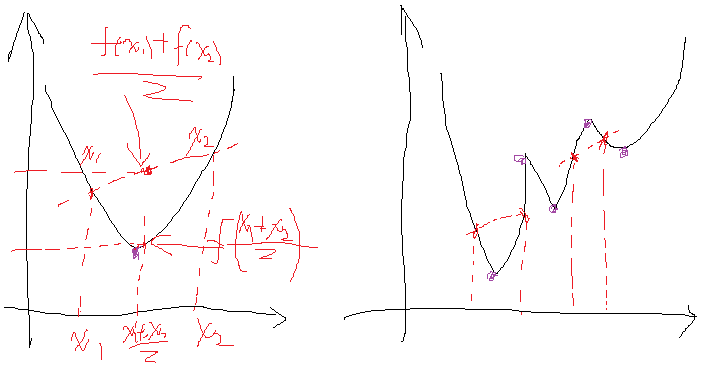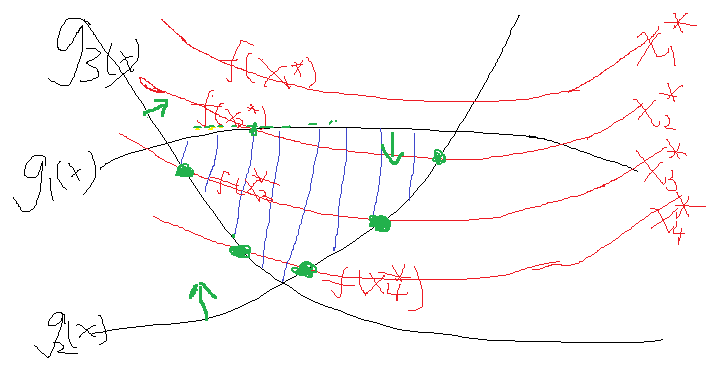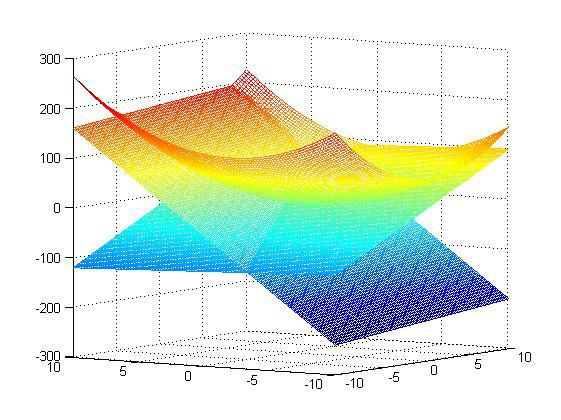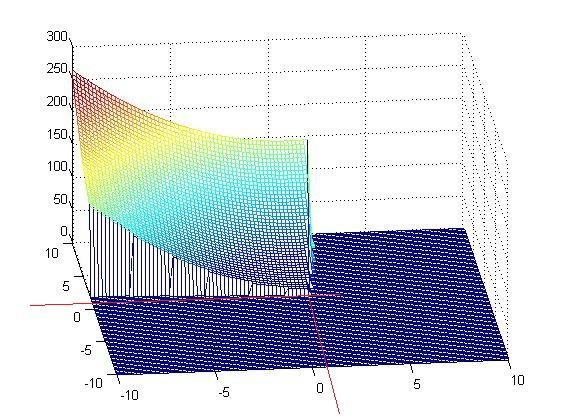# 解密SVM系列（一）：关于拉格朗日乘子法和KKT条件

### （一）关于拉格朗日乘子法

minf=2x21+3x22+7x23s.t.2x1+x2=12x2+3x3=2f(x1)+f(x2)2>f(x1+x22)f(x1)+f(x2)2<f(x1+x22)

minf=2x21+3x22+7x23+α1(2x1+x21)+α2(2x2+3x32)

fx1=4x1+2α1=0x1=0.5α1fx2=6x2+α1+2α2=0x2=α1+2α26fx3=14x3+3α2=0x3=3α214

### （二）关于KKT条件

minf=x212x1+1+x22+4x2+4s.t.x1+10x2>1010x110x2<10

s.t.10x110x2<010x1x210<0

L(x,α)=f(x)+α1g1(x)+α2g2(x)=x212x1+1+x22+4x2+4+α1(10x110x2)+α2(10x1x210)

L(x,α,β)=f(x)+αigi(x)+βihi(x)

(1) L对各个x求导为零；
(2) h(x)=0;
(3) αigi(x)=0αi0$\sum\alpha_ig_i(x)=0，\alpha_i\ge0$αigi(x)=0αi0$\sum\alpha_ig_i(x)=0，\alpha_i\ge0$

minf=x212x1+1+x22+4x2+4s.t.10x110x2<010x1x210<0

L(x,α)=x212x1+1+x22+4x2+4+α1(10x110x2)+α2(10x1x210)

Lx1=2x12α1+10α2=0x1=0.5(α110α2+2)Lx2=2x2+410α1α2=0x2=0.5(10α1+α24)

α1g1(x)=α1(10x110x2)=0α2g2(x)=α2(10x1x210)=0

（1）α1=α2=0$\alpha_1=\alpha_2=0$，那么看上面的关系可以得到x1=1,x2=1$x_1 = 1,x_2=-1$,再把两个x带到不等式约束，发现第一个就是需要满足（10-1+20=29<0)显然不行，29>0的。舍弃

（2）g1(x)=g2(x)=0$g_1(x)=g_2(x)=0$，带进去解得，x1=110/101;x2=90/101,再带回去求解α1α2$\alpha_1，\alpha_2$，发现α1=58/101α2=4/101$\alpha_1=58/101，\alpha_2=4/101$，它们满足大于0的条件，那么显然这组解是可以的。

（3）其他两种情况再去讨论发现是不行的。

x1=110/101=1.08;x2=90/101=0.89,那么它得到结果对不对呢？这里因为函数简单，可以在matlab下画出来，同时约束条件也可以画出来，那么原问题以及它的约束面画出来就如下所示：